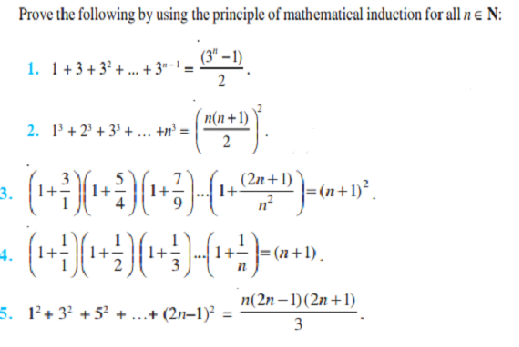# Principle of Mathematical Induction Class 11 Notes - Chapter 4

## What is Mathematical Induction?

Mathematical induction is a specialized form of working on different cases and coming up with observations. Induction is the compilation from a particular set of facts. This method is used to prove a wide range of statements in which we analyze the legitness of the case. The set should be denumerable in order for mathematical induction to work with an infinite set, meaning that it should be having a one-to-one correspondence between the elements of the set in question and the set of positive integers.

In other words, it must be possible to express the set in the form an implied list of discrete elements such as {1, 2, 3, 4, …}.

### Properties of Mathematical Induction

Mathematical induction has to follow statements with respect to the properties they obey:

• When for the value of n is true for statements such as n ≥ 5, we should initiate with satisfying the value n=5 i.e. P(5)
• If the statement provided is true for n=k, and if it satisfies the value n=k then it will also satisfy n=k+1. In order to prove the trueness of the statement, we have to prove n=k+1.

### Inductive Hypothesis

The step mentioned above is the assumption of the trueness of the statement n=k and is referred to as the inductive step or inductive hypothesis.

Let us take an example of the following pattern:

$1=1^{2}=1$ $4=2^{2}=1+3$ $9=3^{2}=1+3+5$ $16=4^{2}=1+3+5+7$

Here we can witness the sum of the first two odd natural numbers is square of the second number which is natural and the pattern continues.

P(n)=$1+3+5+7+11..+(2n-1)=n^{2}$

And P(1) is satisfied, then, it is the first step and the value will satisfy for all natural numbers.

### Important Questions: